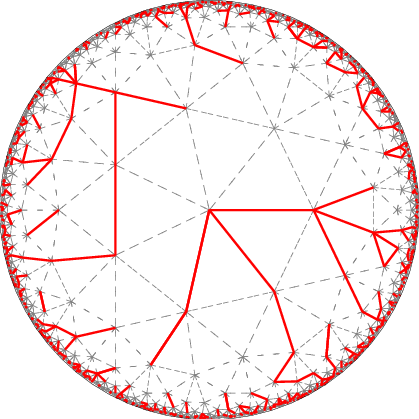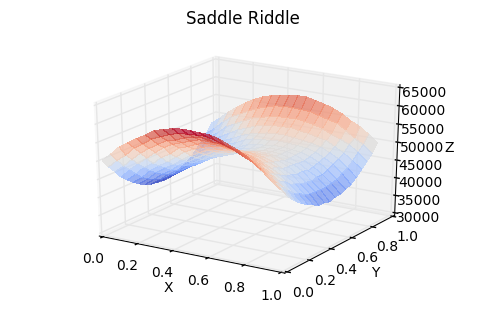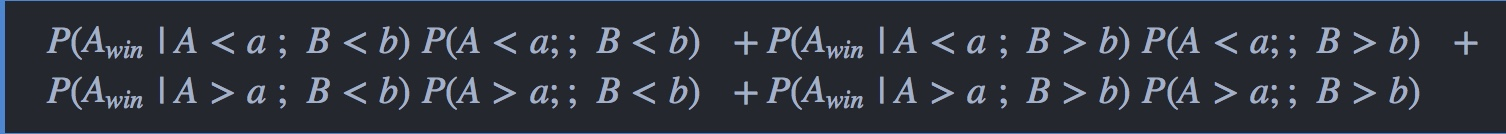# link..linkPoint Curry DiscoVisual Search Engine!# Golden Saddle Problem

Posted on Fri 04 November 2016 in misc

Nothing excites me more than a good mathematical puzzle. Even if I don't end up solving one, I always come out richer in the process by improving the way I think. Combinatorial problems in particular are my favourite.

So you can imagine my excitement when I came across this really interesting probability / game theory riddle recently, courtesy Peter Norvig's Ipython notebooks on probability. Delightful reads these notebooks are, where concepts and popular paradoxes in Probability are introduced using Python.

### Problem Statement¶

Two players go on a hot new game show called “Higher Number Wins.” The two go into separate booths, and each presses a button, and a random number between zero and one appears on a screen. (At this point, neither knows the other’s number, but they do know the numbers are chosen from a standard uniform distribution.) They can choose to keep that first number, or to press the button again to discard the first number and get a second random number, which they must keep. Then, they come out of their booths and see the final number for each player on the wall. The lavish grand prize — a case full of gold bullion — is awarded to the player who kept the higher number. Which number is the optimal cutoff for players to discard their first number and choose another? Put another way, within which range should they choose to keep the first number, and within which range should they reject it and try their luck with a second number?

This is how I approached this problem to begin with.

• Does such a cut-off exist at all?

• If it indeed exists, is it possible to prove that before proceeding to find it?

• Are there multiple cut-offs?

• What's wrong with the trivial solution 0.5?

I had a really hard time convincing myself why 0.5 is not a solution to this problem. On further thinking I realized that if both the cut-offs are equal to any real number in the interval (0,1), the probability of any player winning the game would equal 0.5.

So there might be nothing special about 0.5 after all. It is however the cut-off at which your expected value is maximum and equals 0.625. But maximum expected value isn't the name of the game here. What we need is a cut-off value that maximizes the probability of winning. To put it in simpler terms, if one of the players were to choose 0.5 as a cutoff and play this game a million times, the average value of the score he/she would've kept would be approximately equal to 0.625. This however doesn't ensure that the player would've won most of the times.

Anyway, in the spirit of Peter's notebook, I decided to run a simulation and see if I can spot something curious instead of breaking my head the traditional way.

The idea is to carry out simulations to figure out how the chances of one of the players winning the challenge change with varying cutoffs. In the code below, runsim(a, b) returns the number of times Player 1 wins out of 100,000 simulations if Player 1's cutoff is a and Player 2's cutoff is b. We run this simulation for various values of a and b, plot the results and see if something interesting shows up.

In :
import random
from sympy import *
import numpy as np
init_printing()

def simula(a,b):
trial = (random.random(), random.random())
if trial > a and trial > b:
return 1 if trial > trial else 0
if trial > a and trial < b:
return 1 if trial > random.random() else 0
if trial < a and trial > b:
return 1 if random.random() > trial else 0
if trial < a and trial < b:
return  1 if random.random() > random.random() else 0

def runsim(a,b):
score = 0
for i in range(100000):
score += simula(a,b)
return score


That was easy wasn't it? Took about 20 lines of code in all. (Eventually, I would model my algebraic solution also along the same lines. So yes, not only did this simulation clear things up for me but it also helped me come up with a solution!)

Notice how I avoided equality conditions in the code above. The way I understood the question, if the number on the screen is less than the cut-off, the player is going to give it an other shot and if it is greater than that, he/she is going to settle with the score. But what about the case when the score is exactly equal to the cut-off? I avoided answering this question and proceeded ahead, justifying myself that the 'measure' of these cases is equal to zero. Also, may be the author of the problem meant a player will keep his/her score if it is less than or equal to the cut-off score. As it turns out, this 'boundary condition' was exploited to give a really elegant solution to the problem by the author!

Let's play around with the simulation and see if we can find something interesting.

But first of all, let's see how accurately our simulation represents the game. We can start with the fact that the probability of A winning the game is equal to 0.5, if the cut-offs are equal.

In :
[runsim(i,i) for i in np.arange(0,1.1,0.1)]

Out:
[49857, 50052, 50100, 49839, 50065, 50041, 49739, 50129, 49917, 50081, 49971]

Looks good. Let's verify one more thing just to make sure.

Consider the case of player A choosing a cut-off of 0.5 and player B not choosing one at all. What is the probability of A winning the game?

$P(A_{win})=P(A_{win}\;|\; A_{score}<\frac{1}{2})\;P(A_{score}<\frac{1}{2}) \;\;+\;\; P(A_{win}\;|\; A_{score}>\frac{1}{2})\;P(A_{score}>\frac{1}{2})$

$=\;\;\frac{1}{2}\frac{1}{2}+\frac{1}{2}\frac{1+\frac{1}{2}}{2}\;\;=\;\;\frac{5}{8}\;\;=\;\;0.625$

In :
runsim(0.5,1.61834) #Choose b to be any number greater than 1

Out:
$$62489$$

That's less than 0.1% error from what the theory predicted. The simulation does appear to represent the game faithfully.

When I started working on this problem, it wasn't very clear to me why 0.5 isn't the solution to the problem. Now that I ran the simulation and started exploring the data, I finally managed to grasp the essence of the problem.

The goal of the problem is to find a real number in the interval [0,1] such that, whatever be the cut-off deployed by the opponent, the probability of you winning the game is at least 0.5.

If your opponent is as smart as you are, he/she would've adopted the same strategy and settled down for the same cut-off that you have arrived at. So, assuming the worst, it is in your best interests to find this optimum cut-off.

Let us now try to explore the data. The $10 * 10$ matrix below gives a nice bird's eye view of the numbers, obtained by running the simulation 10 million times. For example, the probability of A winning the game if his cut-off is 0.4 and the opponent's cut-off is 0.7 is equal to the entry in the $4^{th}$ row and $7^{th}$ column of the matrix divided by 100,000. Notice how the elements in the matrix with respect to the diagonal, stack up nicely to reflect the fact that $runsim(a,b) + runsim(b,a) = 100000$

In :
p = [[runsim(j,i) for i in np.arange(0.1,1.1,0.1)]
for j in np.arange(0.1,1.1,0.1)]

In :
Matrix(p)

Out:
$$\left[\begin{matrix}49741 & 46145 & 43094 & 41637 & 41098 & 41406 & 43187 & 45945 & 49606 & 54549\\53901 & 50014 & 46786 & 44521 & 43960 & 44486 & 45898 & 48501 & 52586 & 57750\\56724 & 52999 & 50056 & 47686 & 46620 & 46701 & 48049 & 50907 & 54812 & 60537\\58411 & 55231 & 52305 & 49909 & 48357 & 48562 & 49743 & 52258 & 56575 & 61998\\58852 & 55871 & 53390 & 51710 & 49842 & 49732 & 50422 & 52900 & 56621 & 62719\\58460 & 55446 & 53428 & 51673 & 50624 & 49796 & 50548 & 52768 & 56588 & 61954\\56696 & 54116 & 51762 & 50054 & 49691 & 49127 & 50018 & 51838 & 55281 & 60428\\53990 & 51212 & 48972 & 47489 & 46787 & 47388 & 48194 & 49843 & 53072 & 58178\\50385 & 47126 & 44952 & 43407 & 42807 & 43393 & 44618 & 47133 & 49935 & 54637\\45520 & 41916 & 39596 & 38201 & 37385 & 38194 & 39517 & 42023 & 45518 & 49800\end{matrix}\right]$$

Notice how the $5^{th}, 6^{th}, 7^{th}$ rows, corresponding to Player 1 choosing 0.5, 0.6 and 0.7 for cut-offs respectively, don't have a single entry that is less than 49,000. Similarly, notice how the $5^{th}, 6^{th}, 7^{th}$ columns, corresponding to Player 2 choosing 0.5, 0.6 and 0.7 for cut-offs respectively don't have a single entry greater than 51,000. Drilling down further, making the data more granular, I figured the optimal cut-off is somewhere near 0.6

Let's plot this data and see if we can find something interesting.

In :
import matplotlib.pyplot as plt
from mpl_toolkits.mplot3d import Axes3D
from matplotlib import cm
%matplotlib inline

x = y = np.arange(0.0, 1.0, 0.05)
X, Y = np.meshgrid(x, y)
zs = np.array([runsim(x,y) for x,y in zip(np.ravel(X), np.ravel(Y))])
Z = zs.reshape(X.shape)

fig = plt.figure()
ax = fig.add_subplot(111, projection='3d')         # set the 3d axes
ax.plot_surface(X, Y, Z,
rstride=1,
cstride=1,
alpha=0.7,            # transparency of the surface
cmap=cm.coolwarm,
linewidth=0,
antialiased=False)         # colour map

ax.set_xlabel('X')
ax.set_xlim(0, 1)
ax.set_ylabel('Y')
ax.set_ylim(0, 1)
ax.set_zlabel('Z')
ax.set_zlim(30000, 65000)

ax.set_title('Saddle Riddle', va='bottom')

ax.view_init(elev=25, azim=-58)           # elevation and angle
ax.dist=12                                # distance

plt.show()Sweet. We have what looks like a Saddle Point Graph! It was at this point I realize this is a Game Theory problem with the saddle point describing the equilibria.

It turns out that the optimum cut-off is approximately equal to .61834. To be precise, it is equal to $\phi-1$, where $\phi$ is equal to the golden ratio! How cool is that!

## Algebraic Solution¶

If $a$ and $b$ are the cut-offs chosen by the players $A$ and $B$ respectively, and we manage to derive a function $f(a,b)$ that outputs the probability of $A$ winning the game, we are good to go. We can then examine $f(a,b)$, do some mathemagickery and answer all the questions above.

Assuming $a$ < $b$ without any loss of generality, the required equation is equal to$=\;\;ab/2 + a(1-b)^2/2 + b(1-a^2)/2 + (1-b)^2/2$

Substitute $a$ for $b$ in the above equation and the function becomes a constant equal to $\frac{1}{2}$. Nice.

Finding that much sought after optimum cut-off is now equivalent to finding the saddle points to the above equation. We will use Sympy library to do that.

In :
a,b = symbols('a b')
x = a*b/2 + a*(1-b)**2/2 + b*(1-a**2)/2 + (1-b)**2/2

In :
factor(x)

Out:
$$- \frac{1}{2} \left(a^{2} b - a b^{2} + a b - a - b^{2} + b - 1\right)$$
In :
z = [diff(x,a), diff(x,b)]

In :
z

Out:
$$\left [ - a b + \frac{b}{2} + \frac{1}{2} \left(- b + 1\right)^{2}, \quad - \frac{a^{2}}{2} + \frac{a}{2} \left(2 b - 2\right) + \frac{a}{2} + b - \frac{1}{2}\right ]$$
In :
w = solve(z,[a,b])

In :
simplify(w)

Out:
$$\left ( - \frac{1}{2} + \frac{\sqrt{5}}{2}, \quad - \frac{1}{2} + \frac{\sqrt{5}}{2}\right )$$